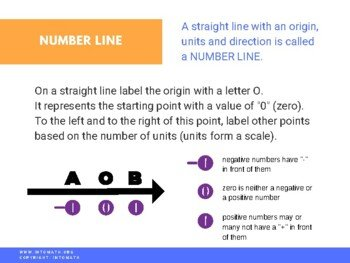CA$2.00 In order to display positive and negative numbers we often use a number line. A number line is a line that contains all negative and positive integers, including zero (the origin). One of the real life examples of a number line is a thermometer. A thermometer has a scale that usually goes from -50 to +50 degrees Celsius. 0 degrees is the middle between the coldest and the hottest temperatures. When we add and subtract positive and negative integers, we can always use a number line to calculate the absolute distance between the two digits – in mathematics this distance is called “absolute value”.Grade 7 Workbook (22 pages with answers) CA$4.00

A number line is an excellent tool that helps understand operations with integers visually.

For example, what is -8 + 9?

We would locate the -8 on the number line and move 9 digits in the positive direction (from left to right). We would land on 1, which would be the answer to the problem.

A number line can also be used to compare positive and negative integers.